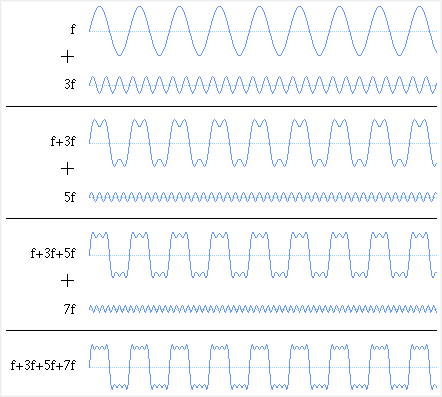# 4. Square Wave Harmonics

Home | Next

A square wave can be constructed from multiple sine waves at different frequencies. The sine waves added in addition to the fundamental frequency are called harmonics; a square wave has harmonics at odd multiples of the fundamental frequency. As higher harmonics are added, the result gets closer to an ideal square wave, which contains infinite harmonics.

```    sin(angle) + sin(3*angle)/3 + sin(5*angle)/5 + sin(7*angle)/7 + ...
```Square wave built from sum of sine waves